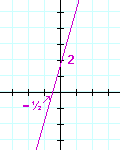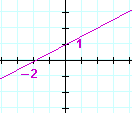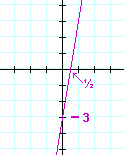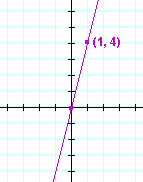# Drawing the graph

Back to Section 1

The x- and y-intercepts of a graph

Drawing the graph

The form  y = ax.

Vertical and horizontal lines

The x- and y-intercepts of a graphThe x-intercept of a graph is that value of x where the graph crosses the x-axis.  The y-intercept is the value of y where it crosses the y-axis.

To find the x-intercept, we have to find the value of x where y = 0 -- because at every point on the x-axis, y = 0.

Example 1.   Calculate the value of x when y = 0, that is, find the x-intercept of  y = 2x + 10.

Solution.   On putting  y = 0, we have to solve the equation,

 2x + 10 = 0. We have: 2x = −10 x = −5.

The x-intercept is −5.

Problem 10.   Calculate the value of x when y = 0.

 a) y = 2x + 4 b) y = 3x − 12 2x + 4 = 0 3x − 12 = 0 2x = −4 3x = 12 x = −2 x = 4
 c) y = 4x + 1 4x + 1 = 0 4x = −1 x = −¼

To find the y-intercept of a graph, we must find the value of y when x = 0 -- because at every point on the y-axis, x = 0.  But when the equation has the form

y  =  ax + b,

the y-intercept is simply b.   When x = 0,

y  =  0 + b  =  b.

Drawing the graph

Two points determine a straight line.  And the two most important points are the x- and y-intercepts.  Therefore whenever we draw a graph, we always mark those points.

Problem 11.

 a) When we have the equation of a straight line, how do we find the x−intercept?

Put y =0 and solve for x.

b)  How do we find the y−intercept?

Put x =0 and solve for y.

Problem 12.   Mark the x- and y-intercepts, and draw the graph of

y = 2x + 6.The x-intercept −3 is the solution to 2x + 6 = 0. The y-intercept is the constant term, 6. The graph is the straight line that passes through those two intercepts.

Example 2.   Mark the x- and y-intercepts, and draw the graph of

5x − 2y  =  10.

Solution.   Although this does not have the form  y = ax + b, the strategy is the same.  Find the intercepts by putting x -- then y -- equal to 0.

 x y y-intercept 0 −5 2 0 x-intercept

When we put x = 0, we have

 −2y = 10. This implies y = −5.

When we put y = 0, we have

 5x = 10. This implies x = 2.

Here is the graph:Problem 13.

 a) In an equation  y = ax + b , where on the graph do we find b?

As the y-intercept.

 b) Where do we find the solution to  ax + b = 0 ?

As the x-intercept.

Problem 14.   Mark the x− and y−intercepts, and draw the graph.

 a) y = 2x − 6 b) y = −3x + 3c) y = 4x + 2 d) x − y = 3See Example 2. e) x − 2y + 2 = 0 f) 2x − 3y − 6 = 0g) y = −x + 1 h) y = 6x − 3Problem 15.   The form  y = ax.

 a) When an equation has the form  y = ax  (for example,  y = 2x), what number is b ?   0
 b) Therefore, what number is the y-intercept?   0

c)   Through which point does the line pass?

The origin. In other words the x- and y- intercepts coincide.

d)   How do we find another point on the line?

Choose any value for x. The equation will then determine the value of y.

(In the next Lesson, we will see that a is the slope of the line.

Example 3.   Draw the graph of  y = 2x.

Solution.   Since b = 0, the graph passes through the origin.  To find another point, let x = 1 for example. Then y = 2 · 1 = 2.  The point (1, 2) is on the graph:Example 4.    Draw the graph of  y  = 23 x.

Solution.   Again, since b = 0, the graph passes through the origin.  To find another point, choose an integer value for x in such a way that y also will be an integer.  Choose x equal to the denominator, 3.  The point (3, 2) then is on the graph.Problem 16.   Draw each graph.

 a) y = 4x b) y = −xc) y = 35 x d) y = − 25 xExample 6.   Vertical and horizontal lines.

An equation of the form

x  =  A number

is the equation of a vertical line.  For example,

x  =  3.x = 3 -- the x-coördinate is 3 -- is true of every point on that line.

An equation of the form

y  =  A number

is the equation of a horizontal line.  For example,

y  =  −4.The y-coördinate is −4 at every point on that line.

Problem 17.   Draw each graph.

 a) x = 5 b) x = −2c) y = 3 d) y = −1Problem 18.

a)   What is the equation of the x-axis?

y = 0

b)   What is the equation of the y-axis?

x = 0Back to Section 1

Next Lesson:  The slope of a straight line

Please make a donation to keep TheMathPage online.
Even \$1 will help.